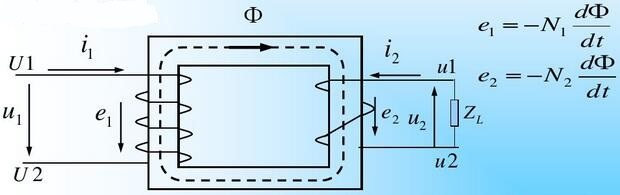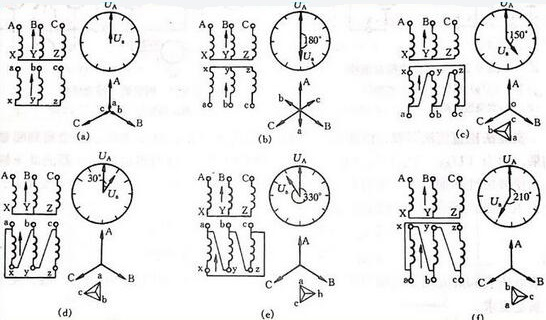## Are Step-Up and Step Down Transformers Interchangeable?

In electricity, step-up transformers increase the voltage of the incoming current, while step-down transformers decrease the incoming current's voltage. So are they interchangeable?

Introduction
Can a step-down transformer be used as a step-up transformer? This involves not only the principle of the transformer, but also the specific components and their functions in circuit. In terms of working principle, the transformer can step down and step up. Does this mean they can be converted? But it is worth noting that the voltage grade, impedance characteristics, impedance voltage characteristics and winding current, etc. all determine whether the step-down transformer can be used for step-up. So here we will explain it in detail.

1 Electrical Transformer Working Principle
Transformer is a common electrical equipment that can be used to transform a certain value of alternating voltage into another one with the same frequency. A step-up transformer is a device used to transform a low alternating voltage into another higher value with the same frequency. While the step-down transformer is a very important equipment in the power transmission and transformation system. That is, its normal operation is not only related to its own safety and reliable power supply for users, but also directly affects the stability of the power system.
Transformers generally have two functions, one is the buck-boost function, and the other is the impedance matching function. Let me talk about the former. Usually we use a variety of voltages in applications. For example, the life lighting power is 110V, the industrial safety lighting is 36V, and the voltage of the welding machine needs to be adjusted. These are inseparable from the transformer. For example, according to the principle of mutual inductance, the transformer passes through the main and auxiliary coils to reduce the voltage to the voltage we need.EMF Formula

The main parts of the transformer are the iron core and the windings on it. The two windings are only magnetically coupled but not electrically connected. Add an alternating voltage to the primary winding to generate alternating magnetic flux that links the primary and secondary windings, and induce the electromotive force (EMF) in the two windings respectively. As long as the number of turns of the primary and secondary windings is different, the purpose of voltage transformation can be achieved by transformer.

2 Differences between Step-Down Transformer and Step-Up Transformer
1) The step-down transformer converts the higher voltage at the input of the power supply into a lower voltage for our normal use to achieve the purpose of step-down.
2) The step-up transformer can convert a low voltage into a higher voltage. (Additionally, the inverter transformer is also a kind of step-up transformer).
In principle, the step-down transformer and the step-up transformer are the same, the specific difference is the inductance, copper consumption, and winding capacity of the high-voltage side and the low-voltage side. The same transformer, no matter it is used for step-up or step-down, the iron loss is the same. Under no-load conditions, the high-voltage side winding of the step-down transformer has many turns, large impedance, large inductance, small current and low copper loss, in addition, the high-voltage side winding has a larger capacity. At this time, it becomes a step-up transformer, the iron loss is the same, but the low-voltage side winding has a small number of turns and a small impedance. The inductance is small and the copper loss is small, and the primary side capacity is smaller than the secondary at this time.
But there is a question. When the step-down transformer is converted to a step-up transformer, can the rated parameters of the low-voltage side coil withstand the loss under no-load conditions? If so, how much power is left for the high-voltage side.
Whether to increase or decrease voltage depends on the ratio of the number of turns of the primary coil and the secondary coil. 1:1 is only for isolation. Therefore, the step-down transformer can be used as a step-up transformer, but it may not work in practice.Electrical Transfomer Structure

3 Example Analysis
As above mentioned, step-up transformer and step-down transformer cannot be used as a reverse conversion. Because the step-up transformer is equivalent to stepping up low-voltage power into high-voltage power. For the system, its low-voltage side is equivalent to absorbing electric energy, and the high-voltage side sending electric energy is equivalent to the power source. That is, the load of the system accepts the standard rated voltage, and the voltage output on the power supply side takes into account the voltage drop of the circuit and the transformer itself, about 10%. In order to ensure that the voltage delivered to the user is exactly the rated voltage, the voltage output on the high voltage side is 10% higher than the rated voltage.
For example, if the rated voltage of the low-voltage side of a step-up transformer is 20kV and the high-voltage side is 110kV, the receiving voltage of the low-voltage side is 20kV, and the high-voltage side is 10% higher, about 121kV. If you consider the transformation ratio, suppose that the low-voltage side has 20 turns, and the high-voltage side cannot be 110 turns but 121 turns. If this step-up transformer is used as a step-down transformer, its high-voltage side can be regarded as a load from the system and can only receive a rated voltage of 110kV, and meanwhile the output voltage of the low-voltage side cannot reach 20kV, which can’t work normally. Similarly, the step-down transformer cannot be used as a step-up transformer. In the actual application process, the structure and protection part of the step-down transformer is different from that of the step-up. So this action will slowly reduce the stability of the transformer and may affect its service life.
Of course, there is also a case where a step-down transformer can be used as a step-up one, as long as the voltage does not exceed the primary and secondary voltage.Electrical Transformer Parts

4 Theoretical Analysis
Nowadays, it is very common that the voltage instability fluctuates during our usual mains electricity use. Therefore, each family needs to install a power supply device for its own power line. Considering that some people often use low voltage, and some people's home voltage is always high, so there are step-up transformers and step-down transformers.
We first look at the rectifier transformer. We found that the secondary wire on its surface is particularly thick, which is due to the larger current in the secondary circuit. It can be imagined from this that if the secondary circuit is used as the primary side, its impedance must be very small, and the power supply must provide a large current to obtain the required voltage on the secondary side of the transformer, resulting in low conversion efficiency. Ordinary transformers do have this possibility. For example, the electric energy generated by the user's self-provided low-voltage generator may pass the power transformer (step-down) back to the grid. So once the self-provided generator starts, you need to open the circuit breaker connected to the grid. Even with this possibility, it is not arbitrarily that the electric energy can be fed back to the grid through the transformer.
Let's look at the expression of AC voltage: . Note that U on the right side of the equal sign is the effective value of the voltage, and this voltage must meet the specified rated value, f is the frequency (which must also meet the condition of the standard value), and Φ is the phase difference.
We call these three parameters on the primary side of the transformer consistent with the grid requirements on the secondary side of the transformer, which is called synchronous operation. It is a necessary operation that must be performed for the power supply and the power grid to be combined. And the same period value must fully comply with the specific specification value given by the specification standard.
Since the synchronization parameters of the power grid are fixed, the generator must adjust its own synchronization value. The adjustment process of the same period is not very easy. The synchronous period can only be satisfied in an instant. We can only achieve as close as possible, that is, quasi-synchronous. If it is found that the quasi-synchronization is completed, immediately close the circuit breaker, and the electric energy generated by the generator can be boosted by the transformer and sent to the grid. It can be seen that this is not easy, and it can only be achieved by supporting a synchronous measuring instrument or a relay.
Pay attention to the wiring problem of the transformer, that is, the connection group of the transformer. Generally, the phase of the high-voltage side of the transformer is deviated from the low-voltage side. Standards and specifications are vividly expressed using a clock. For example, Y11 and Y0, respectively indicate the connection at 11 o'clock and 0 o'clock (11 o'clock means that the difference between the two is 30 degrees in electrical angle, and 0 o'clock has no deviation). Therefore, when doing synchronous operations, we must also consider what time the transformer wiring is. In U.S, many households have solar power generation devices as auxiliary power supplies to generate electricity for own use. When the electricity is enough, it can be fed back to the grid and get benefit. Obviously, there are synchronization devices and power transformers here.Phase Deviation

Search
Categories